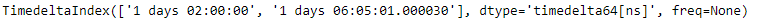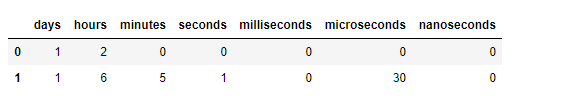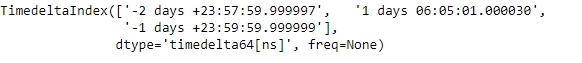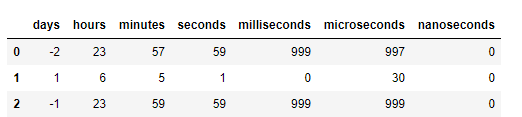Python | Pandas TimedeltaIndex.components

• Last Updated : 29 Dec, 2018

Python is a great language for doing data analysis, primarily because of the fantastic ecosystem of data-centric python packages. Pandas is one of those packages and makes importing and analyzing data much easier.

Pandas TimedeltaIndex.components attribute return a dataframe of the components (days, hours, minutes, seconds, milliseconds, microseconds, nanoseconds) of the Timedeltas.

Syntax: TimedeltaIndex.components

Return : a DataFrame

Example #1: Use TimedeltaIndex.components attribute to return a dataframe of the components of the Timedeltas.

 # importing pandas as pdimport pandas as pd  # Create the TimedeltaIndex objecttidx = pd.TimedeltaIndex(data =['1 days 02:00:00',                                 '1 days 06:05:01.000030'])  # Print the TimedeltaIndexprint(tidx)

Output :Now we want to return a dataframe of the components of the tidx object.

 # return a dataframe constructed from# the components of the Timedeltatidx.components

Output :As we can see in the output, the TimedeltaIndex.components attribute has returned a dataframe constructed from the components of the Timedelta

Example #2: Use TimedeltaIndex.components attribute to return a dataframe of the components of the Timedeltas.

 # importing pandas as pdimport pandas as pd  # Create the TimedeltaIndex objecttidx = pd.TimedeltaIndex(data =['-1 days 2 min 3us', '1 days 06:05:01.000030',                                                 '-1 days + 23:59:59.999999'])  # Print the TimedeltaIndexprint(tidx)

Output :Now we want to return a dataframe of the components of the tidx object.

 # return a dataframe constructed from# the components of the Timedeltatidx.components

Output :As we can see in the output, the TimedeltaIndex.components attribute has returned a dataframe constructed from the components of the Timedelta

My Personal Notes arrow_drop_up